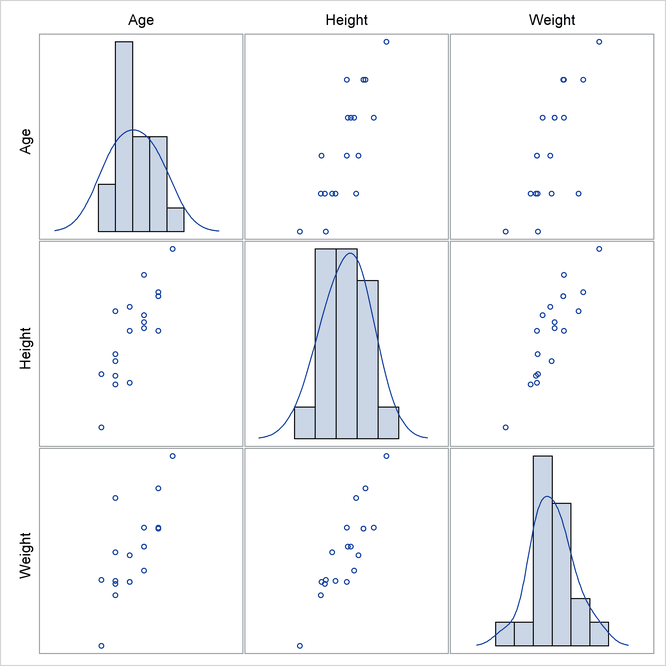# Statistical Graphics Using ODS

### The SGSCATTER Procedure

You can use the SGSCATTER procedure to produce scatter plot matrices. The following step creates a scatter plot matrix from all of the numeric variables in the Class data set (available in the Sashelp library) and produces Figure 21.62:

proc sgscatter data=sashelp.class;
matrix _numeric_ / diagonal=(kernel histogram);
run;


The diagonal cells of Figure 21.62 contain a histogram and a kernel density fit. The off-diagonal cells contain all pairs of scatter plots.

Figure 21.62: Scatter Plot Matrix with PROC SGSCATTERThe MATRIX statement creates a symmetricscatter plot matrix. Other statements are also available. The PLOT statement creates a panel that contains one or more individual scatter plots. The COMPARE statement creates a rectangularscatter plot matrix. Linear and nonlinear fits can be added, and many graphical features can be requested with options.